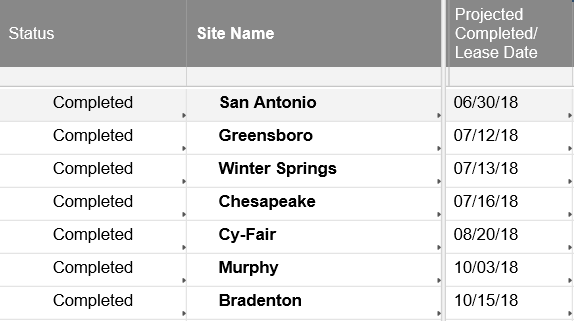# COUNTIF formula

I am trying to count the number of projects completed between July 1, 2018 and June 30, 2019 with this formula:

=COUNTIFS({Status}, ="Completed", {Completion Date}, >=DATE(2018, 7, 1) <= DATE(2019, 6, 30))

The outcome is supposed to be 6, but it is reading back 7 (it is also counting San Antonio).  Does anyone know why??• Thank you! This worked